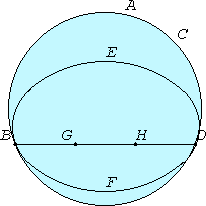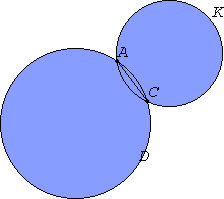# Proposition 13

A circle does not touch another circle at more than one point whether it touches it internally or externally.

For, if possible, let the circle ABDC touch the circle EBFD, first internally, at more points than one, namely D and B.III.1

Take the center G of the circle ABDC and the center H of EBFD.

III.11

Therefore the straight line joined from G to H falls on B and D.

Let it so fall, as BGHD.

Then, since the point G is the center of the circle ABCD and BG equals GD, therefore BG is greater than HD. Therefore BH is much greater than HD.

Again, since the point H is the center of the circle EBFD, BH equals HD, but it was also proved much greater than it, which is impossible.Therefore a circle does not touch a circle internally at more points than one.

I say further that neither does it so touch it externally.

For, if possible, let the circle ACK touch the circle ABDC at more points than one, namely A and C. Join AC.

Then, since on the circumference of each of the circles ABDC and ACK two points A and C have been taken at random, the straight line joining the points falls within each circle, but it fell within the circle ABCD and outside ACK, which is absurd.

Therefore a circle does not touch a circle externally at more points than one.

And it was proved that neither does it so touch it internally.

Therefore a circle does not touch another circle at more than one point whether it touches it internally or externally.

Q.E.D.

## Guide

In the second impossible figure there are three curves connecting A to C. The two circles are not supposed to cut each other, but just to touch each other at the two points A and C, and the straight line AC should lie between the two circles and not within either one.

There are logical flaws in this proof similar to those in the last two proofs.

This proposition is not used in the rest of the Elements.